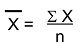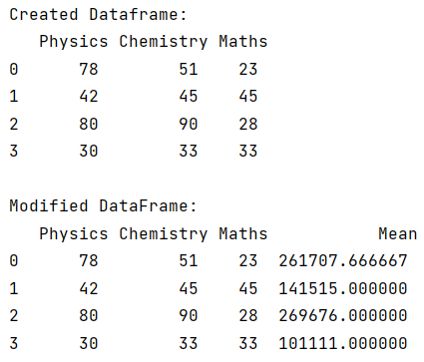# Compute row average in pandas

Given a Pandas DataFrame, we have to compute row average.
Submitted by Pranit Sharma, on July 28, 2022

Pandas is a special tool that allows us to perform complex manipulations of data effectively and efficiently. Inside pandas, we mostly deal with a dataset in the form of DataFrame. DataFrames are 2-dimensional data structures in pandas. DataFrames consist of rows, columns, and data.

The most fascinating key point about pandas is that it contains numerous amounts of function to calculate almost everything mathematically and logically.

With the help of pandas, we can calculate the mean of any column in a DataFrame, the column values should be integer or float values and not string.

To compute the row average in pandas, we are going to use df.mean().

We use pandas.DataFrame.mean(axis=0) directly to calculate the average value of row.

The average of a particular set of values is the sum of all the values divided by the total number of values.

Mathematically, it can be represented as:Let us understand with the help of an example,

## Python code to compute row average

```# Importing Pandas package
import pandas as pd

# Creating a Dictionary
d = {
'Physics': ['78', '42', '80', '30'],
'Chemistry': ['51', '45', '90', '33'],
'Maths': ['23', '45', '28', '33']
}

# Creating a dataframe
df = pd.DataFrame(d)

# Display Original dataframe
print("Created Dataframe:\n",df,"\n")

# Calculating mean
df['Mean'] = df.mean(axis=1)

# Display modified DataFrame
print("Modified DataFrame:\n",df)
```

Output:Languages: » C » C++ » C++ STL » Java » Data Structure » C#.Net » Android » Kotlin » SQL
Web Technologies: » PHP » Python » JavaScript » CSS » Ajax » Node.js » Web programming/HTML
Solved programs: » C » C++ » DS » Java » C#
Aptitude que. & ans.: » C » C++ » Java » DBMS
Interview que. & ans.: » C » Embedded C » Java » SEO » HR
CS Subjects: » CS Basics » O.S. » Networks » DBMS » Embedded Systems » Cloud Computing
» Machine learning » CS Organizations » Linux » DOS
More: » Articles » Puzzles » News/Updates